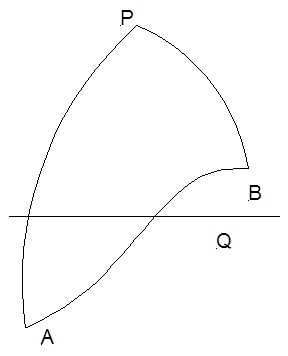What is meaning of Vertex of Great Circle ? |

# What is meaning of Vertex of Great Circle ?

This is the point on the great circle that is nearest to the pole. At this point the latitude is maximum. To every great circle, there are two vertices one in the northern hemisphere VN and one in the southern hemisphere VS, unless great circle is the equator. Vertex is noted as latitude and longitude.

In triangle PAV, PA and initial course A are known. d.long APV needs to be determined to find the longitude and PV needs to be determined to find the latitude.

###### Using Napier’s Rule:     sin mid part = product of cos of opposite parts

sin PV                          =      cos (co – PA)      x       cos (co – A)

sin PV                          =       cos lat A            x       sin A

cos lat V                    =       cos lat A             x       sin V

 cos lat V                               =             cos lat A               x              sin A
###### Using Napier’s Rule:

sin mid part = product of tan of adjacent parts

sin (co – PA)             =       tan (co – P)       x       tan (co – A)

cos PA                          =       cotan P    x       cotan A

tan P                            =                          1

sin lat A x       tan A